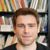## Julia Community 🟣

melonedo

Posted on • Updated on

# Julia Interpolation Cookbook

This is a repost of the one on my own blog

Metaprogramming is one of the key features of Julia, apart from multiple dispatching. While interpolation is essential to metaprogramming in Julia, it is usually but not always intuitive, as some tricky cases are hard to search for. this post covers some of the tricky cases when interpolating exressions that I encounter.

## Official manual

Although interpolation saves you from directly manupulating `Expr` objects that represent Julia AST, you are still expected to understand the AST underneath that Julia really understands, and to parse `Expr` input (MLStyles.jl and MacroTools.jl may help). In fact I often think in AST to decide what to do and code in interpolation, which is more readable.

This article only covers some corner cases, so it is expected that you read the Program representation and the Expressions and evaluation sections in the official manual about metaprogramming before reading the content below. Also, the article about Julia ASTs in the manual is a good reference when you compose `Expr` objects by hand.

## How to do splatting interpolation?

In short, use `\$(exprs...)`. But this isn't really that easy. You must make sure splatting is interpolated in the right context, since the interpolated expressions are the same in every context.

For example, suppose `exprs = (:a, :b, :c)`, `:(\$(exprs...))` will result in syntax error because Julia does not understand what you want. Below is some common use cases:

``````julia> exprs = (:a, :b, :c)
(:a, :b, :c)

julia> :(\$(exprs...);) # list of expressions
quote
a
b
c
end

julia> :(\$(exprs...),) # tuple
:((a, b, c))

julia> :(; \$(exprs...)) # named tuple. (;a,b,c) is short for (a=a,b=b,c=c)
:((; a, b, c))

julia> :[\$(exprs...)] # vector
:([a, b, c])

julia> :[\$(exprs...);] # also vector
:([a; b; c])

julia> :[\$(exprs...);;] # row vector (matrix)
:([a;; b;; c])
``````

Note currently keyword arguments (and named tuple) can not be constructed with interpolation unless you use the short form above, so to construct a general keyword argument, you must use the `Expr` way:

``````julia> f = :(foo(1,2))
:(foo(1, 2))

julia> insert!(f.args, 2, Expr(:parameters, Expr(:kw, :a, 3)));

julia> f
:(foo(1, 2; a = 3))
``````

## How to include a literal `Symbol` in quotes?

``````julia> :(\$(Meta.quot(:a)) + b)
:(:a + b)
# or
julia> :(\$(QuoteNode(:a))+b)
:(:a + b)
``````

They do result in different ASTs, but the difference is not significant for a single symbol.

## How to include a `\$` in quotes?

The "uninterpolated" quote `\$a` as in `:(\$a + b)` is valid input for macros, but is not documented. Let's examine what it is first:

``````julia> a=1
1

# does not work
julia> :(\$a)
1

julia> macro dump1(ex) ex end
@dump1 (macro with 1 method)

# because `\$a` is not valid expression alone
julia> @dump1 \$a
ERROR: syntax: "\$" expression outside quote around REPL:1
Stacktrace:
[...]

julia> macro dump2(ex) dump(ex) end
@dump2 (macro with 1 method)

julia> @dump2 \$a
Expr
args: Array{Any}((1,))
1: Symbol a
``````

So we see that `\$a` is `Expr(:\$, :a)`.